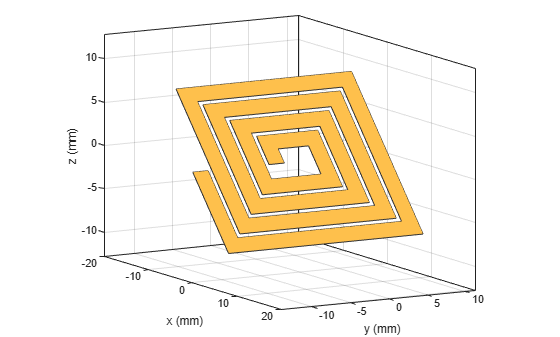# rotateY

Rotate RF PCB shape about y-axis and angle

## Syntax

``c = rotateY(shape,angle)``

## Description

example

````c = rotateY(shape,angle)` rotates a shape by the specified angle about the y-axis.```

## Examples

collapse all

Create and display a spiral trace.

```trace = traceSpiral; show(trace)```Rotate the spiral trace by 45 degrees about the y-axis.

`traceRotY = rotateY(trace,45);`

Display the rotated shape. Set the camera line of sight to display in 3-D space.

```show(traceRotY) view(60,10)```## Input Arguments

collapse all

RF PCB shape created using custom elements and shape objects of RF PCB Toolbox™, specified as an object.

Example: `shape = bendCurved;` specifies the shape as a `bendCurved` object.

Angle of rotation, specified as a scalar in degrees.

Example: `45` rotates the shape around the y-axis by 45 degrees.

Data Types: `double`

## Version History

Introduced in R2021b